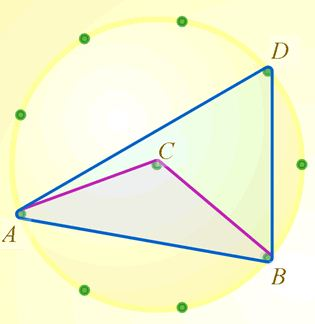# Geometry

Sites with clear resources by topic – Geometry

Geoboard Activities

From Nrich comes an interactive Geoboard and a selection of problems to introduce circle theorems. As with all Nrich resources teachers’ notes are included. See these on the Subtended Angles problem for example.From Ben Sparks on GeoGebra – interactives for all 8 circle theorems discussed at GCSE maths.

On the subject of Circle Theorems, see also Tim Devereux’s GeoGebra appletsYou can access each theorem from the menu on the left which includes a useful summary of all the theorems. From the menu, we can see that proofs are also available.

GCSE Content – Geometry
Properties and constructions
1. use conventional terms and notations: points, lines, vertices, edges, planes, parallel lines, perpendicular lines, right angles, polygons, regular polygons and polygons with reflection and/or rotation symmetries; use the standard conventions for labelling and referring to the sides and angles of triangles; draw diagrams from written descriptions

2. use the standard ruler and compass constructions (perpendicular bisector of a line segment, constructing a perpendicular to a given line from/at a given point, bisecting a given angle); use these to construct given figures and solve loci problems; know that the perpendicular distance from a point to a line is the shortest distance to the line

3. apply the properties of angles at a point, angles at a point on a straight line, vertically opposite angles; understand and use alternate and corresponding angles on parallel lines; derive and use the sum of angles in a triangle (e.g. to deduce and use the angle sum in any polygon, and to derive properties of regular polygons)

4. derive and apply the properties and definitions of: special types of quadrilaterals, including square, rectangle, parallelogram, trapezium, kite and rhombus; and triangles and other plane figures using appropriate language

5. use the basic congruence criteria for triangles (SSS, SAS, ASA, RHS)

6. apply angle facts, triangle congruence, similarity and properties of quadrilaterals to conjecture and derive results about angles and sides, including Pythagoras’ Theorem and the fact that the base angles of an isosceles triangle are equal, and use known results to obtain simple proofs

7. identify, describe and construct congruent and similar shapes, including on
coordinate axes, by considering rotation, reflection, translation and enlargement
(including fractional and negative scale factors)

8. describe the changes and invariance achieved by combinations of rotations, reflections and translations

9. identify and apply circle definitions and properties, including: centre, radius, chord, diameter, circumference, tangent, arc, sector and segment

10. apply and prove the standard circle theorems concerning angles, radii, tangents and chords, and use them to prove related results

11. solve geometrical problems on coordinate axes

12. identify properties of the faces, surfaces, edges and vertices of: cubes, cuboids, prisms, cylinders, pyramids, cones and spheres

13. construct and interpret plans and elevations of 3D shapes

Mensuration and calculation
14. use standard units of measure and related concepts (length, area, volume/capacity, mass, time, money, etc.)

15. measure line segments and angles in geometric figures, including interpreting maps and scale drawings and use of bearings

16. know and apply formulae to calculate: area of triangles, parallelograms, trapezia; volume of cuboids and other right prisms (including cylinders)

17. know the formulae: circumference of a circle = 2πr = πd, area of a circle = πr2 ; calculate: perimeters of 2D shapes, including circles; areas of circles and composite shapes; surface area and volume of spheres, pyramids, cones and composite solids

18. calculate arc lengths, angles and areas of sectors of circles

19. apply the concepts of congruence and similarity, including the relationships between lengths, areas and volumes in similar figures

20. know the formulae for: Pythagoras’ theorem, a2 + b2 = c2, and the trigonometric ratios, sinθ , cosθ and tanθ

21. know the exact values of sinθ and cosθ for θ = 0°, 30°, 45°, 60° and 90°; know the exact value of tanθ for θ = 0° , 30°, 45°and 60°

22. know and apply the sine rule and cosine rule to find unknown lengths and angles

23. know and apply Area = ½ absin C to calculate the area, sides or angles of any triangle.

Vectors
24. describe translations as 2D vectors

25. apply addition and subtraction of vectors, multiplication of vectors by a scalar, and diagrammatic and column representations of vectors; use vectors to construct geometric arguments and proofs

Resources by topic – Geometry

Exemplification Examples